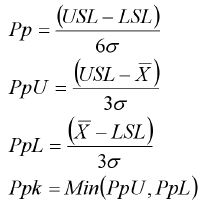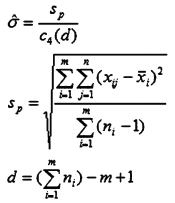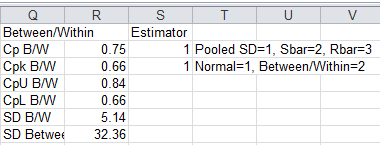# Cp Cpk Formulas versus Pp Ppk Formulas

## Formulas for Cp Cpk and Pp Ppk are Similar

People often get confused about the difference between Pp/Ppk and Cp/Cpk.

### Cp, Cpk Formula & Calculations(Cpk > 1.33 is desirable)

## σ̂

### Pp, Ppk Formula & Calculations(Ppk > 1.33 is desirable)

## σ

### Sigma Estimator Calculation - Three Different Methods

#### Pooled Standard Deviation

Use when n > 2#### Average of the Subgroup Standard Deviations

Use when n > 4
c4 is a constant based on subgroup size
Sbar = Σ(σi)/n#### Average of the Subgroup Ranges

Use when n = 1 to 4
d2 is a constant based on subgroup size
Rbar = Average(Ri) (Average of the Ranges in samples)n = the subgroup size

### Thinking About Trying to Do This Yourself? Don't - Here's Why:### TIME

You will probably spend hours trying to figure it out.

Why not download a QI Macros free trial and start doing it correctly immediately?### COMPLEXITY

You can try to do the calculations yourself, but if you are like most folks, you'll make an error.

There are several formulas to choose from and lots of places to make mistakes.### CUSTOMERS

Chances are you are not doing this for the sheer fun of it.

If you're providing these results to customers shouldn't you use a tool that's proven and reliable?

### QI Macros Calculations are Tested and Accurate

QI Macros works right in Excel. It uses these defaults and provides the option to change methods if required:

• Pooled Standard Deviation when n >= 2 and
• Rbar/d2 when n = 1.Users can change the sigma estimator calculation after they have run a histogram by changing the estimator fields in cells S2 and S3 on the far right of the histogram data sheet.

In addition between changing from Pooled Standard deviation to Sbar or Rbar, users can also choose Between/Within Deviation.Note: Minitab started using Pooled Standard Deviation to calculate Cp/Cpk, and control limits on XbarR and XbarS charts in versions 15 and 16. Minitab 17 went back to Rbar/d2 and Sbar/c4 for XbarR/S control limits, but retained pooled stdev for Cp/Cpk calculations when using multiple samples.

### USL & LSL = Nominal Value (+)(-) Tolerance

To calculate your Upper and Lower Specification Limits, provide your Nominal Value (+)(-) Tolerance (USL = Nominal Value + Tolerance, LSL = Nominal Value - Tolerance).

Once you have inputted your USL & LSL into the template, input your data - your Process Capability Metrics will then start calculating automatically (a minimum of 2 data values are required).

### One-Sided or Unilateral Specification Limits

Use CpU (USL) or CpL (LSL) for Cpk.

### Pp and Ppk Calculations in Weibull Histogram

Unlike the Pp and Ppk calculations which rely on a Normal distribution, the Weibull histogram uses the WEIBULL (or WEIBULL.DIST) function to calculate the Z-scores for the USL/LSL and from these it calculates Pp and Ppk. There is no Cp or Cpk calculation possible in the Weibull Histogram.

Pp = (PpU + PpL) / 2

Ppk = Min (PpU, PpL)

PpU = NORMSINV (WEIBULL (USL, Shape, Scale, TRUE))

PpL = NORMSINV (WEIBULL (LSL, Shape, Scale, TRUE))

If there is only a USL or LSL, the Weibull histogram Ppk is either PpU or PpL.### QI Macros Tools that Calculate Cp, Cpk and Pp, Ppk

• Histogram - calculates Cp, Cpk, Pp, Ppk and 20 other metrics using your data and spec limits.
• Weibull Histogram - calculates Pp, Ppk and other metrics using your data and spec limits.
• Capability Suite - creates six charts including histogram, control charts, probability plot, values plot and capability plot. Also calculates Cp, Cpk and Pp, Ppk.
• Cp Cpk Template - calculates Cp, Cpk and Pp, Ppk on multiple sets of data.

### Why Choose QI Macros to Perform Capability Analysis and Draw Histograms?### Fast and Easy to Use

• Works Right in Excel
• Calculates Cp Cpk Pp Ppk
• Accurate No-Worry Results
• Free Training Anytime### Proven and Trusted

• 100,000 Users in 80 Countries
• Celebrating 20+ Years
• Five Star CNET Rating - Virus Free### Affordable

• Only \$349 USD
Quantity Discounts Available
• No annual fees
• Free Technical Support